Function Repository Resource:

# LinearlyIndependent

Determine whether a set of vectors is linearly independent

Contributed by: Wolfram|Alpha Math Team
 ResourceFunction["LinearlyIndependent"][{vect1,vect2,…}] returns the conditions under which the given vectors are mutually linearly independent.

## Details

For numeric input, ResourceFunction["LinearlyIndependent"] will return True or False. For symbolic input, the result may be a ConditionalExpression.

## Examples

### Basic Examples (4)

Test some two-dimensional vectors for linear independence:

 In:=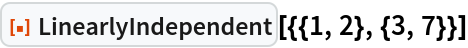Out=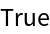Test some three-dimensional vectors for linear independence:

 In:=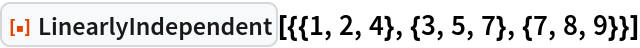Out=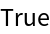This set of vectors is linearly dependent:

 In:=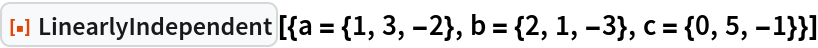Out=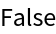Confirm that the third vector can be written as a linear combination of the first two:

 In:=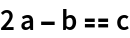Out=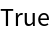LinearlyIndependent works with any number of vectors of any dimension:

 In:=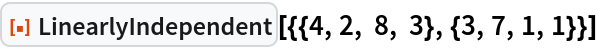Out=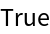### Scope (3)

For vectors with symbolic parameters, LinearlyIndependent may return a ConditionalExpression:

 In:=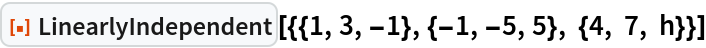Out=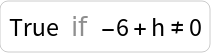In:=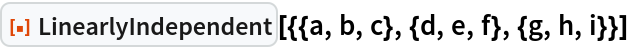Out=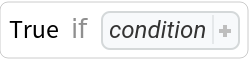A True/False result may be obtained by giving values to the parameters:

 In:=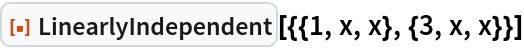Out=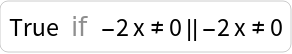In:=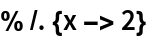Out=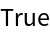LinearlyIndependent accepts vectors with complex components:

 In:=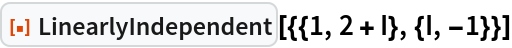Out=### Properties and Relations (6)

A set of vectors is linearly independent if and only if the rank of the row matrix composed of the vectors equals the length of the vectors:

 In:=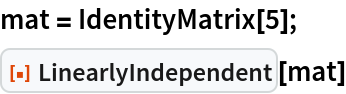Out=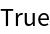In:=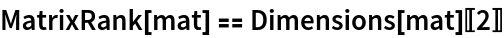Out=A set of vectors is linearly independent if and only if the rank of the row matrix composed of the vectors has a zero-dimensional null space:

 In:=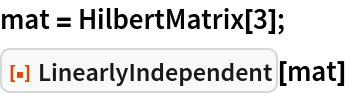Out=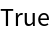In:=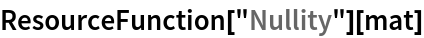Out=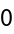Or, alternatively:

 In:=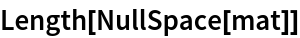Out=A set of vectors is linearly independent if and only if the rank of the row matrix composed of the vectors has a nonzero determinant:

 In:=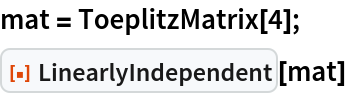Out=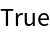In:=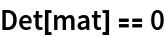Out=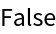A set of vectors is linearly independent if and only if its row-reduced form has a no zeros along its diagonal:

 In:=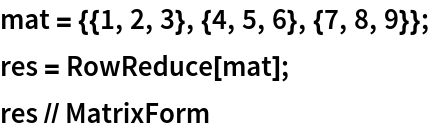Out=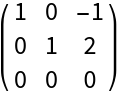In:=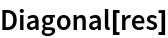Out=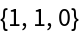In:=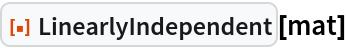Out=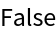The zero vector is linearly dependent on every other vector:

 In:=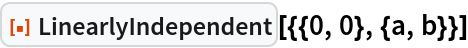Out=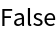LinearlyIndependent will not evaluate if the vectors do not all have the same length:

 In:=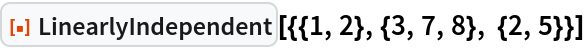Out=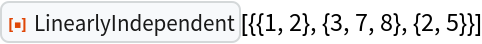## Publisher

Wolfram|Alpha Math Team

## Version History

• 3.0.0 – 23 March 2023
• 2.1.0 – 11 May 2021
• 2.0.0 – 24 January 2020
• 1.0.0 – 03 October 2019